## Helmholtz Differential Equation

A Partial Differential Equation which can be written in a Scalar version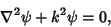(1)

or Vector form,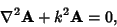(2)

where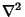is the Laplacian. When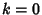, the Helmholtz differential equation reduces to Laplace's Equation. When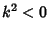, the equation becomes the space part of the diffusion equation.The Helmholtz differential equation can be solved by Separation of Variables in only 11 coordinate systems, 10 of which (with the exception of Confocal Paraboloidal Coordinates) are particular cases of the Confocal Ellipsoidal system: Cartesian, Confocal Ellipsoidal, Confocal Paraboloidal, Conical, Cylindrical, Elliptic Cylindrical, Oblate Spheroidal, Paraboloidal, Parabolic Cylindrical, Prolate Spheroidal, and Spherical Coordinates (Eisenhart 1934). Laplace's Equation (the Helmholtz differential equation with) is separable in the two additional Bispherical Coordinates and Toroidal Coordinates.

If Helmholtz's equation is separable in a 3-D coordinate system, then Morse and Feshbach (1953, pp. 509-510) show that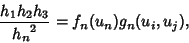(3)

where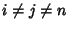. The Laplacian is therefore of the form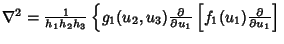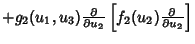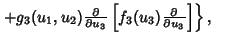(4)
which simplifies to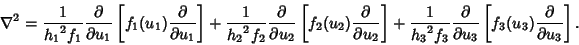(5)

Such a coordinate system obeys the Robertson Condition, which means that the Stäckel Determinant is of the form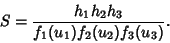(6)

 Coordinate System Variables Solution Functions Cartesian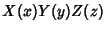exponential, Circular Functions, Hyperbolic Functions Circular Cylindrical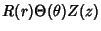Bessel Functions, Exponential Functions, Circular Functions Conical Ellipsoidal Harmonics, Power Ellipsoidal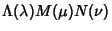Ellipsoidal Harmonics Elliptic Cylindrical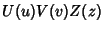Mathieu Function, Circular Functions Oblate SpheroidalLegendre Polynomial, Circular Functions Parabolic Bessel Functions, Circular Functions Parabolic Cylindrical Parabolic Cylinder Functions, Bessel Functions, Circular Functions Paraboloidal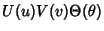Circular Functions Prolate SpheroidalLegendre Polynomial, Circular Functions Spherical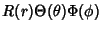Legendre Polynomial, Power, Circular Functions

See also Laplace's Equation, Poisson's Equation, Separation of Variables, Spherical Bessel Differential Equation

References

Eisenhart, L. P. Separable Systems in Euclidean 3-Space.'' Physical Review 45, 427-428, 1934.

Eisenhart, L. P. Separable Systems of Stäckel.'' Ann. Math. 35, 284-305, 1934.

Eisenhart, L. P. Potentials for Which Schroedinger Equations Are Separable.'' Phys. Rev. 74, 87-89, 1948.

Morse, P. M. and Feshbach, H. Methods of Theoretical Physics, Part I. New York: McGraw-Hill, pp. 125-126 and 509-510, 1953.